## Saturday, 9 August 2014

### CHAPTER 3 - Worked Out Examples

 Example: 1
 Find the middle term(s) in the expansion of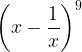${\left( {x - \dfrac{1}{x}} \right)^9}$
 Solution: 1
Since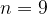$n = 9$, there will be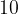$10$ terms in the expansion, which means that there will be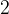$2$ middle terms in the expansion, the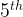${5^{th}}$ and the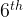${6^{th}}$: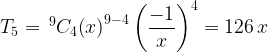${T_5} = {\,^9}{C_4}{\left( x \right)^{9 - 4}}\left( {\dfrac{{ - 1}}{x}} \right)_{}^4 = 126\,x$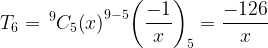${T_6} = {\,^9}{C_5}{\left( x \right)^{9 - 5}}{\left( {\dfrac{{ - 1}}{x}} \right)_5} = \dfrac{{ - 126}}{x}$
 Example: 2
 Is there any term in the expansion of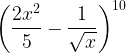${\left( {\dfrac{{2{x^2}}}{5} - \dfrac{1}{{\sqrt x }}} \right)^{10}}$ that will be independent of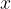$x$?
 Solution: 2
The general term in the expansion is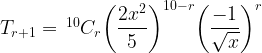${T_{r + 1}} = {\,^{10}}{C_r}{\left( {\dfrac{{2{x^2}}}{5}} \right)^{10 - r}}{\left( {\dfrac{{ - 1}}{{\sqrt x }}} \right)^r}$$= {\,^{10}}{C_r}{\left( {\dfrac{2}{5}} \right)^{10 - r}}{\left( { - 1} \right)^r}\,\,{x^{20 - 2r - \dfrac{r}{2}}}$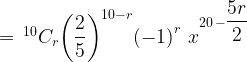$= {\,^{10}}{C_r}{\left( {\dfrac{2}{5}} \right)^{10 - r}}{\left( { - 1} \right)^r}\,\,{x^{20\, - \dfrac{{5r}}{2}}}$
Thus, for the term that is independent of$x$, we have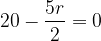$20 - \dfrac{{5r}}{2} = 0$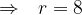$\Rightarrow \,\,\,\, r = 8$
Thus, the term free of$x$ is the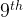${9^{th}}$ term given by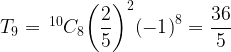${T_9} = {\,^{10}}{C_8}{\left( {\dfrac{2}{5}} \right)^2}{\left( { - 1} \right)^8} = \dfrac{{36}}{5}$
 Example: 3
 Evaluate the sum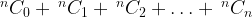$^n{C_0} + {\,^n}{C_1} + {\,^n}{C_2} + \ldots + {\,^n}{C_n}$.
 Solution: 3
We have already evaluated this sum in the chapter on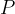$P$ &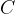$C$. That approach was as follows: this sum basically counts the number of all sub-groups of a set of size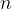$n$; this can also be counted by focusing on each element of the set, which has two corresponding choices – you either include it into your sub-group or you don’t, which means that the total number of ways to form sub-groups is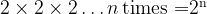${\rm{2 \times 2 \times 2 }}\ldots n\,{\rm{ times = }}{{\rm{2}}^{\rm{n}}}$. The sum of the binomial coefficients therefore equals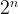${2^n}$.
Here, we evaluate the same sum using a binomial approach. Consider the following expansion:${\left( {1 + x} \right)^n} = \,{\,^n}{C_0} + {\,^n}{C_1}x + {\,^n}{C_2}\,{x^2} + {\,^n}{C_3}\,{x^3} + \ldots + {\,^n}{C_n}\,{x^n}$
If we put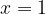$x = 1$, we simply obtain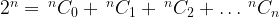${2^n} = {\,^n}{C_0} + {\,^n}{C_1} + {\,^n}{C_2} +\ldots {\,^n}{C_n}$
Thus, the same result is obtainable from both a combinatorial and a binomial approach.
We can also derive another useful result by putting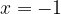$x = - 1$ in the above relation, so that we obtain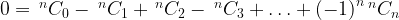$0 = {\,^n}{C_0} - {\,^n}{C_1} + {\,^n}{C_2} - {\,^n}{C_3} + \ldots + {\left( { - 1} \right)^n}{\,^n}{C_n}$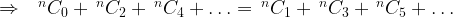$\Rightarrow \,\,\,\, { ^n}{C_0} + {\,^n}{C_2} + {\,^n}{C_4} + \ldots = {\,^n}{C_1} + {\,^n}{C_3} + {\,^n}{C_5} +\ldots$
This states the sum of the even-numbered coefficients is equal to the sum of the odd-numbered coefficients. Can you prove this using a combinatorial approach?
As an exercise, prove the following relations: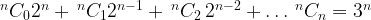$^n{C_0}{2^n} + {\,^n}{C_1}{2^{n - 1}} + {\,^n}{C_2}\,{2^{n - 2}} + \ldots {\,^n}{C_n} = {3^n}$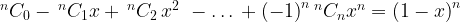$^n{C_0} - {\,^n}{C_1}x + {\,^n}{C_2}\,{x^2}\,\, - \ldots \, + {\left( { - 1} \right)^n}{\,^n}{C_n}{x^n} = {\left( {1 - x} \right)^n}$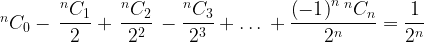$^n{C_0} - \,\dfrac{{^n{C_1}}}{2} + \,\dfrac{{^n{C_2}}}{{{2^2}}}\, - \dfrac{{^n{C_3}}}{{{2^3}}} + \ldots \, + \dfrac{{{{\left( { - 1} \right)}^n}{\,^n}{C_n}}}{{{2^n}}} = \dfrac{1}{{{2^n}}}$